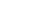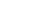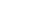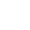# 學生與政府的「墨西哥對峙」僵局｜囚徒困局系列(三)

2014/03/26 | | 標籤：（轉載自nilnimest 的部落格

• A，B：參賽者
• C，D：策略，C=合作 (Cooperate)，D=不合作 (Defect)
• (D，C)，(C，C)，(D，D)，(C，D)：結果或策略組合
• T：收益，參賽者一方合作另一方不合作時，不合作者的誘惑 (Temptation)
• R：收益，參賽者雙方均合作時，合作者的報酬 (Reward)
• P：收益，參賽者雙方均不合作時，不合作者的懲罰 (Punishment)
• S：收益，參賽者一方合作另一方不合作時，合作者的傻瓜收益 (Sucker’s Payoff)
• 4=最好收益; 3=次好收益; 2=次壞收益; 1=最壞收益

「囚徒困局」 (Prisoner’s Dilemma)

 Player B C D Player A C R=3，R=3 S=1，T=4 D T=4，S=1 P=2，P=2

T>R>P>S

「懦夫賽局」 (Chicken)

 Player B C D Player A C R=3，R=3 S=2，T=4 D T=4，S=2 P=1，P=1

T>R>S>P

「懦夫賽局」的原型故事是兩人開車對撞，如果選擇在相撞前避開，就是合作 (C)，否則就是不合作 (D)。因為避開被視為「懦夫」 (Chicken)，故賽局俗稱「懦夫賽局」。一般人應該都會同意當懦夫至少比撞死好，所以 T>R>S>P 是成立的。冷戰時期的古巴飛彈危機事件中，美蘇之間核子戰爭一觸即發，但最後關頭蘇聯避開了；賽局的最後結果是理性選擇理論所預測的一個納許均衡的結果。

 學生 和平離開立法院 堅持佔領立法院 政府 不驅離 R=3，R=3 S=2，T=4 驅離 T=4，S=2 P=1，P=1

 學生 和平離開行政院 堅持佔領行政院 政府 不驅離 R=3，R=3 S=1，T=4 驅離 T=4，S=1 P=2，P=2《可能性調查署2》上線囉！接下來每周都會有新影片，快去按讚訂閱開啟小鈴鐺

## 關於作者